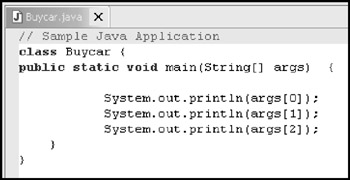Passing Arguments to Java

Java Applications define an array of optional String arguments. The example shown in Figure 26.9 makes use of arguments. It receives a list of values and prints them:Figure 26.9: Passing arguments to a Java application.

This line of code invokes the Buycar Java class and passes it three arguments "Explorer," "Jeep," and "Accura." The application prints each of the values as follows:

• Explorer

• Jeep

• Accura

Strings are not the only kind of data Java has to work with. The various numeric data types, such as the integer type discussed earlier, are commonly used in Java and are often used in mathematical formulas. A list of valid arithmetic operators are listed in Table 26.2.

 + Add — Subtract * Multiply / Divide % Modulus (remainder after division) += Add to itself -= Subtract from itself *= Times itself /= Divide into itself Var++ Return value of variable then add one to variable ++Var Add one to variable then returns its new value Var−− Return value of variable then subtract one from it −−Var Subtract one from variable then returns its new valueIBM i5/iSeries Primer(c) Concepts and Techniques for Programmers, Administrators, and Sys[... ]ators
ISBN: N/A
EAN: N/A
Year: 2004
Pages: 245